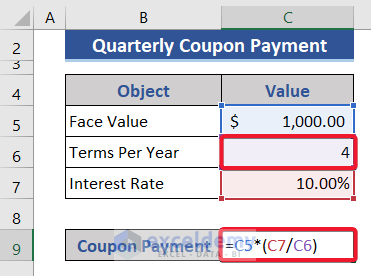# How to Calculate Coupon Payment in Excel (4 Suitable Examples)

Coupons are issued against the bonds. Coupon payments are given based on the interest rate. In this article, we will discuss how to calculate coupon payment in Excel with proper illustration.

## Basic Formula to Calculate Coupon Payment

The coupon payment is the amount of interest given based on the interest rate with the bond. The equation is:Coupon payments are of two types: Fixed coupon payment and variable coupon payment. In the case of fixed coupon payment, the interest rate is fixed all the time. As a coupon payment is also the same. On the other hand, in variable coupon payments, the interest rate changes from time to time. So, coupon payment also changes.

## 4 Examples to Calculate Coupon Payment in Excel

In this article, we will show some examples to calculate coupon payments in Excel. We have taken a dataset of some values of objects.### Example 1: Calculate Yearly Coupon Payment

In this example, we will show how to calculate annual or yearly coupon payments. As we are going to calculate yearly coupon payments, we will get that only one time in a single year. So, the value of terms per year is 1.

📌 Steps:

• Now, we add a new row to represent the coupon payment.• Then, put the following formula on Cell C9.
`=C5*(C7/C6)`• Finally, press the Enter button to get the result.We get yearly coupon payments successfully.

### Example 2: Determine Half-Yearly Coupon Payment

In this example, we will determine the half-yearly coupon payment. That means we will get coupons yearly 2 times.

📌 Steps:

• Copy and paste the following formula on Cell C9.
`=C5*(C7/C6)`Here, the terms per year are 2.

• After that, press the Enter button.Finally, we get the half-yearly coupon payment.

### Example 3: Find Quarterly Coupon Payment

In this example, we will try to find out the quarterly coupon payment. Here, we will get payment 4 times a year. The difference in this example is that the value of terms per year is 4, which means we will get coupon payments in each quarter.

📌 Steps:

• Put the same formula used previously on Cell C9.
`=C5*(C7/C6)`

###• Then, press the Enter button.

### Example 4: Custom Coupon Payment Calculation

In this example, we will calculate custom coupon payments, not like in previous sections yearly, half-yearly, etc.

📌 Steps:

• Here we want to get a coupon payment 6 times a year, which means every 60 days coupon payment will be provided.• Put the following formula on Cell C9.
`=C5*(C7/C6)`• Finally, press the Enter button to get the result.This amount of interest will provide every 60 days interval.

## Conclusion

In this article, we showed 4 examples explaining how to calculate coupon payments in Excel. I hope this will satisfy your needs. Please have a look at our website Exceldemy.com and give your suggestions in the comment box.

## Related Articles#### Alok Paul

Hello, this is Alok. I am working as an Excel & VBA Content Developer at Exceldemy. I want to provide solutions to various Excel-based problems. I completed my study at East West University major in Telecommunications Engineering. I love traveling, reading books, playing cricket.

We will be happy to hear your thoughts5 Excel Hacks You Never Knew